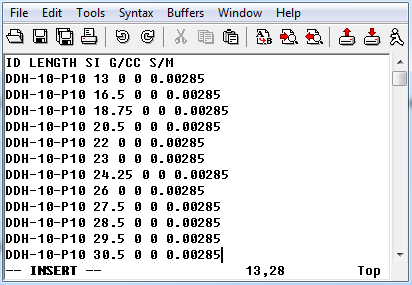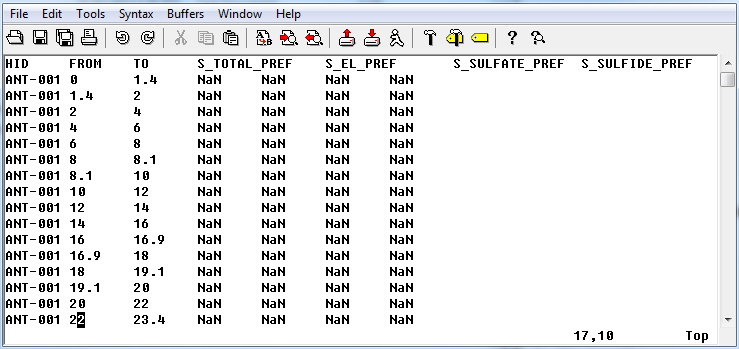# 5.1.12. Borehole property file format¶

GIFtools assumes that the borehole property file is in either the XYZ or CSV file format. This file requires either the length downhole for point measurements or two columns defining the start/end to the observation section. The file may contain multiple properties that would be set in the input/output header later in GIFtools. It is possible to import an integer field that describes geology or characters (e.g., “PK” or “Pyroclastic Kimberlite” if a CSV file). The file must have the at least the following information (but not necessarily these specific names or order):

1. Hole_ID: If the hole ID names require a space, then a CSV file should be used. These identifications can be numeric or characters (with no quotes), but should be consistent with the collar and survey files.

2. Length: Length of observation down the borehole OR

3. Depth_From: Start of the observation section (if length not given)

4. Depth_To: End of the observation section (if length not given)

## 5.1.12.1. Examples¶

### 5.1.12.1.1. Example 1: Length¶

Below is a sample borehole property file (XYZ format) where “ID” is the Hole_ID three properties can be imported (SI, G/CC, and S/M):### 5.1.12.1.2. Example 1: Depth section¶

Below is a sample borehole property file (XYZ format) where “HID” is the Hole_ID and “FROM” is the Depth_From, “TO” is the Depth_to (note NaN are the values of no data):